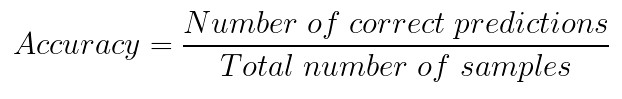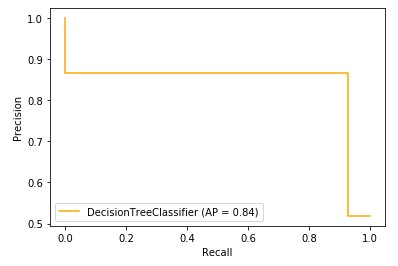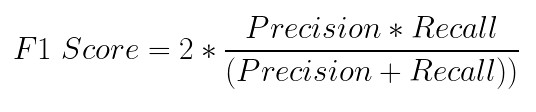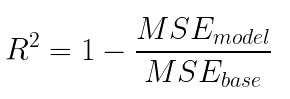Select Page

Thus far in our journey through Machine Learning Basics, we covered several topics. We investigated some regression algorithms, classification algorithms and algorithms that can be used for both types of problems (SVMDecision Trees and Random Forest). Apart from that, we dipped our toes in unsupervised learning, saw how we can use this type of learning for clustering and learned about several clustering techniques.

Finally, in the previous article, we talked about regularization and machine learning model performance. In all these articles, we used Python for “from the scratch” implementations and libraries like TensorFlow, Pytorch and SciKit Learn. Also, we used optimization techniques such as Gradient Descent.

Are you afraid that AI might take your job? Make sure you are the one who is building it.

STAY RELEVANT IN THE RISING AI INDUSTRY! 🖖

What we haven’t mentioned is how we measure and quantify the performance of our machine learning models, ie. which metrics do we use. In this article, we explore exactly that, which metrics can we use to evaluate our machine learning models and how we do it in Python. Before we go deep into each metric for classification and regression algorithms, let’s check out which libraries we need to install for this tutorial.

## Prerequisites

For the purpose of this article, make sure that you have installed the following Python libraries:

• NumPy – Follow this guide if you need help with installation.
• SciKit Learn – Follow this guide if you need help with installation.
• Pandas – Follow this guide if you need help with installation.

Once installed make sure that you have imported all the necessary modules that are used in this tutorial.

``````from math import sqrt
from typing import List
from itertools import combinations

import numpy as np
import pandas as pd

from sklearn import metrics
from sklearn.preprocessing import minmax_scale
from sklearn.model_selection import train_test_split
from sklearn.tree import DecisionTreeClassifier

import matplotlib.pyplot as plt``````

Apart from that, it would be good to be at least familiar with the basics of linear algebra, calculus and probability.

## Classification Metrics

Since the problems we use machine learning fall within different categories, we have different metrics for different types of problems. First, let’s explore metrics that are used for classification problems. In order to represent all these metrics we use simple data:

``````actual_values = [1, 1, 0, 1, 0, 0, 1, 0, 0, 0]
predictions =   [1, 0, 1, 1, 1, 0, 1, 1, 0, 0]``````

So our dataset is composed of two classes – Class 0 and Class 1. The model predicted the class of some samples well, while the others it misclassified.

### Confusion Matrix

There are some basic terms that we need to consider when it comes to the performance of classification models. These terms are best described and defined through the confusion matrix. Confusion matrix or Error Matrix is one of the key concepts when we are talking about classification problems. This matrix is the NxN matrix and it is a tabular representation of model predictions vs. actual values.

Confusion Matrix is for the example data that we use is built like this:Each column and row is dedicated to one class. On one end we have the actual values and on the other end predicted values. What is the meaning of the values in the matrix? In the example above, from 4 values marked as Class 0, our model correctly classified 3 values and misclassified 1 value. This model, from the 6 values marked as Class 1, correctly labeled 3 and misclassified 3. If we refer to the Class 1 as positive and the Class 0 as a negative class, then 3 samples predicted as Class 0 are called as true-negatives, and the 1 sample predicted as Class 1 is referred to as false-negative. The 3 samples correctly classified as Class 1 are referred to as true-positives, and those 3 misclassified instances are called false-positive.Remember these terms, since they are important concepts in machine learning. It is one of the most asked questions in the job interviews. Note that false-positives are also called Type I error and false-negatives are called Type II error. The good news is that we can use Sci-Kit Learn to get this matrix:

``print(metrics.confusion_matrix(actual_values, predictions))``
``````[[3 3]
[1 3]]``````

The result is exactly like in the table above. This matrix is not only giving us details about how our prediction model works but on the concepts laid out in this matrix we are building some of the other metrics. Other classification metrics can be retrieved again using Sci-Kit Learn, or to be more precise using classification report:

``print(metrics.classification_report(actual_values, predictions))``
``````              precision    recall  f1-score   support

0       0.75      0.50      0.60         6
1       0.50      0.75      0.60         4

accuracy                           0.60        10
macro avg       0.62      0.62      0.60        10
weighted avg       0.65      0.60      0.60        10``````

Let’s go into more details for each of them. Note that the support column represents the number of samples of the true response that lie in that class.

### Accuraccy

We start off with the metric that is easies one to understand – accuracy. It is calculated as a number of correct predictions divided by the total number of predictions. When we multiply it by 100 we get accuracy in percents.From the above output, we can see that the accuracy of our predictions is 0.60, meaning 60%. Let’s check that. For the Class 0 model correctly predicted 3 out of 6 and for the Class 1 model correctly predicted 3 out of 4. If we put that into accuracy formula we get (3 + 3) / (6 + 4) = 6 / 10 = 0.6. There is one more way to get this metric:

``print(f'Accuracy Score is: {metrics.accuracy_score(actual_values, predictions) * 100} % ')``
``Accuracy Score is: 60.0 %``

Accuracy however is a tricky metric because it can give us wrong impressions about our model. This is especially the case in the situations where the database is imbalanced, ie. there are many samples of one class and not much of the other. Meaning, if your model is performing well on the class that is dominant in the dataset, accuracy will be high, even though the model might not perform well in other cases. Also, note that models that overfit have an accuracy of 100%.

### Precision

Precision is a very useful metric and it caries more information than the accuracy. Essentially, with precision, we answer the question: “What proportion of positive identifications was correct?”. It is calculated for each class separately with the formula:Its value can go from 0 to 1. Applied to our example, precision for the Class 0 can be calculated as samples of correctly predicted as Class 0 divided by the total number of samples predicted as Class 0 – 3/4 = 0.75. For the Class 1 – 3/6 = 0.5. These values can be seen in the classification report above. We can also calculate precision score like this:

``print(f'Precision Score is: {metrics.precision_score(actual_values, predictions)}')``
``Precision Score is: 0.5``

It goes without saying that we should aim for higher precision.

### Recall

Recall can be described as the ability of the classifier to find all the positive samples. With this metric, we are trying to answer the question: “What proportion of actual positives was identified correctly?” It is defined as the fraction of samples from a specific class which are correctly predicted by the model or mathematically:This metric falls within the 0-1 range as well, with the 1 being the best value. Applied to our example, recall for the Class 0 can be calculated as samples of correctly predicted as Class 0 divided by the total number of samples predicted as Class 0 – 3/6 = 0.5. For the Class 1 – 3/4 = 0.75. These values can be seen in the classification report above. We can also calculate recall score like this:

``print(f'Recall Score is: {metrics.recall_score(actual_values, predictions)}')``
``Recall Score is: 0.75``

Precision and Recall are different and yet so similar. Precision is a measure of result relevancy, while recall is a measure of how many truly relevant results are returned. In the beginning, it might hard to decipher the difference between these two. If you are confused as well, imagine this situation.A client calls a bank in order to verify that her accounts are secure. Bank says that everything is properly secured, however in order to double-check, they ask a client to remember every time that she shared account details with someone. What is the probability that the client will remember every single time she did that? So, the client remembers 10 situations in which that happened, even though in reality there were 8 situations, ie. she falsely identified two additional situations. This means that the client had a recall of 100%, meaning she did cover all the necessary options. However, the client’s precision is 80% since two of those situations are falsely identified as such.

### Precision-Recall Curve

In order to correctly evaluate a model, both metrics need to be taken into consideration. Unfortunately, improving precision typically reduces recall and vice versa. The precision-recall curve shows the tradeoff between precision and recall. To get precision-recall curve we use Sci-Kit Learn function plot_precision_recall_curve with provided estimator:

``disp = metrics.plot_precision_recall_curve(model, X_test, y_test, color='orange')``The area under the curve represents both high recall and high precision. High scores for both show that the classifier is returning accurate results with a majority of positive results. Many other metrics are developed based on the definitions both precision and recall. One of them is the F1 Score.

### F1 Score

F1 Score is probably the most popular metric that combines precision and recall. It represents harmonic mean of them. For binarry classification, we can define it with formula:For our example, we can calculate F1 score for Class 0 as – 2 * 0.5 * 0.75 / (0.5 + 0.75) = 0.6. For Class 1 we get the same value – 0.6. These values can be found in the classification report or running f1_score Sci-Kit Learn Method:

``print('F1 Score:', metrics.f1_score(actual_values, predictions))``
``F1 Score: 0.6``

This formula can be generalized for classification problems with more classes as:where β is 1 in binary classification, ie. it represents the number of possible classes reduced by 1. The best value for F1 score is at 1 and the worst score at 0.

### Receiver Operating Characteristic (ROC) curve & Area Under the curve (AUC)

Ok, those are all metrics from the classification report. However, there are several other techniques that we can apply in order to evaluate the performance of our model. When predicting a class of a sample, the machine learning algorithm first calculates the probability that the processed sample belongs to a certain class and if that value is above some predefined threshold it labels is as that class. For example, for a first sample algorithm predicts that there is a 0.7 (70%) chance that it is Class 0 and threshold is 0.6 the sample will be labeled as Class 0. This means that for different thresholds we can get different labels. This is where we can use the ROC (Receiver Operating Characteristic) curve. This curve shows the true positive rate against the false-positive rate for various thresholds.However, this metric isn’t helping us with model evaluation directly. What is expecially interesting about the image above is the area under the curve or AUC. This metric is, in fact, used as a measure of performance. We can say that ROC is a probability curve and AUC measure the separability, ie. AUC-ROC combination tells us our model capable to distinguish classes. The higher this value the better. We can calculate it fairly easy using Sci-Kit Learn function roc_auc_score:

``print('AUC-ROC:', metrics.roc_auc_score(actual_values, predictions))``
``AUC-ROC: 0.625``

### Log Loss

What is really interesting about this metric is that it is one of the most used evaluation metrics in Kaggle competitions. Log Loss is a metric that quantifies the accuracy of a classifier by penalizing false classifications. Minimizing this function can be, in a way, observed as maximizing the accuracy of the classifier. Unlike the accuracy score, this metric’s value represents the amount of uncertainty of our prediction based on how much it varies from the actual label. For this, we need probability estimates for each class in the dataset. Mathematically we can calculate it as:Where N is the number of samples in the dataset, yi is the actual value for the i-th sample, and yi’ is a prediction for the i-th sample. In code, we can calculate it like this:

``print('LOGLOSS:', metrics.log_loss(actual_values, predictions))``
``LOGLOSS: 13.815750437193334``

This metric is also called logistic loss or cross-entropy loss in the literature.

## Regression Metrics

Since the goal differs when solving regression problems we need to use different metrics. In general, the output of the regression machine learning model is always continuous and thus metrics need to be aligned for that purpose. Just like for classification we use simple data that looks like this:

``````actual_values = [9, -3.3, 6, 11]
predictions =  [8.5, -2.9, 6, 9.2]``````As you can see some predictions deviate from the actual values. Let’s see how we can calculate the quality of these predictions.

### Mean Absolute Error – MAE

As the name suggests this metric calculates the average absolute distance (error) between the predicted and target values. It is defined by the formula:Where N represents the number of samples in the dataset, yi is the actual value for the i-th sample, and yi’ is the predicted value for the i-th sample. This metric is robust to outliers, which is really nice. In the code, we use the mean_absolute_error method:

``print (f'MAE: {metrics.mean_absolute_error(actual_values, predictions)}')``
``MAE: 0.6750000000000002``

### Mean Squared Error – MSE

This is probably the most popular metric of all regression metrics. It is quite simple, it finds the average squared distance (error) between the predicted and actual values. The formula that we use to calculate it is:Where N represents the number of samples in the dataset, yi is the actual value for the i-th sample, and yi’ is the predicted value for the ith sample. The result is a non-negative value and the goal is to get this value as close to zero as possible. This function is often used as a loss function of a machine learning model. In the code, we use the mean_squared_error method:

``print (f'MSE: {metrics.mean_squared_error(actual_values, predictions)}')``
``MSE: 0.9125000000000005``### Root Mean Square Error – RMSE

Another very popular metric. It is a variation of the MSE metric. In general, it shows what is the average deviation in predictions from the actual values and it follows an assumption that error is unbiased and follows a normal distribution. We calculate this value by the formula:Just like MSE, RMSE is a non-negative value, and a value 0 is the value we are trying to achieve. A lower RMSE is better than a higher one. There is no out of the box code for this metric, we can calculate it quite easy using Python:

``````def rmse(actual_values, predictions):
actual_values = np.asarray(actual_values)
predictions = np.asarray(predictions)
return np.sqrt(((predictions - actual_values) ** 2).mean())

print(f'RMSE: {rmse(actual_values, predictions)}')``````
``RMSE: 0.9552486587271403``

Or we can use mean_squared_error again:

``print(f'RMSE: {sqrt(metrics.mean_squared_error(actual_values, predictions))}')``
``RMSE: 0.9552486587271403``

RMSE punishes large errors and is the best metric for large numbers (actual value or prediction). Note that this metric is affected by outliers, so make sure that you remove them from the dataset beforehand.

### Root Mean Squared Logarithmic Error – RMSLE

Another variation on MSE and RMSE is RMSLE. Formula is defined like this:In the code, we can use mean_squared_log_error function. Note that data must be scaled in 0-1 range:

``````actual_values_ranged = minmax_scale(actual_values, feature_range=(0,1))
predictions_ranged = minmax_scale(predictions, feature_range=(0,1))

print(f'RMSLE: {sqrt(metrics.mean_squared_log_error(actual_values_ranged, predictions_ranged))}')``````
``RMSLE: 0.033145260915431275``

RMSLE is robust to the outliers and it penalizes the underestimations more than the overestimations. If the values of the samples are large numbers, consider using RMSE, because this metric is not the best fit for it.### R Squared

The metrics like RMSE and RMSLE are quite useful, however sometimes not intuitive. What we lack is some sort of benchmark for them. In cases where we need a more intuitive approach, we can use the R-Squared metric. The formula for this metric goes as follows:Where MSEmodel is the MSE of the predictions against real values, while MSEbase is the MSE of mean prediction against real values. This means that we use the mean of the predictions as a benchmark. Quite elegant, isn’t it? To calculate it in the code we use r2_score:

``print (f'R Squared: {metrics.r2_score(actual_values, predictions)}')``
``R Squared: 0.9696004330897203``

## Conclusion

In this article, we did a very interesting summary of some of the most popular performance metrics that are used in the machine learning world. We separated these metrics into two big groups and learned the math behind them and how we can calculate them using Sci-Kit Learn and basic Python functionalities.#### Nikola M. Zivkovic

CAIO at Rubik's Code

Nikola M. Zivkovic a CAIO at Rubik’s Code and the author of book “Deep Learning for Programmers“. He is loves knowledge sharing, and he is experienced speaker. You can find him speaking at meetups, conferences and as a guest lecturer at the University of Novi Sad.

Rubik’s Code is a boutique data science and software service company with more than 10 years of experience in Machine Learning, Artificial Intelligence & Software development. Check out the services we provide.

Ultimate Guide to Machine Learning with Python

Everything from Python basics to the deployment of Machine Learning algorithms to production in one place.

Become a Machine Learning Superhero TODAY!